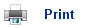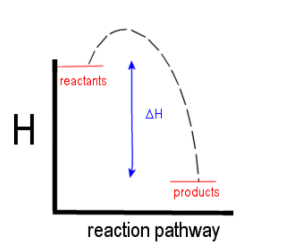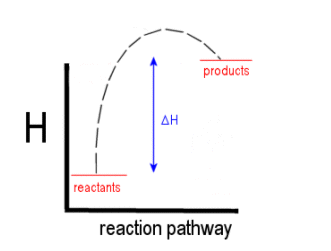# Energy changes and chemical reactions

• Some chemical reactions give out heat, these are called exothermic reactions and have a negative enthalpy change (DH= -ne).
• Other chemical reactions are endothermic, meaning that they take in heat (DH= +ne).
• When a reaction gives out heat, the chemical reactants are losing energy. This energy is used to heat up the surroundings. The products end up with less energy than what the reactants had, but the surroundings end up with more energy.• In an endothermic reaction, the reactants take in energy from the surroundings, resulting in the products having more energy than the reactants.• We call the heat changes associated with chemical reactions enthalpy changes (DH).
• The equation to work out the enthalpy change is

HProuducts – HReactants

• The enthalpy change is defined as the heat exchange with the surroundings at constant pressure, but generally it does not matter about the pressure.
• Enthalpy changes are measured in Kilojoules per mol (KJmol-1).

CH4(g) + 2O2(g) à CO2(g) + H2O(l)        DH= -890 KJ mol-1

• This means that for every one mole of methane that has reacted, 890 KJ are released as heat to the surroundings.
• When calcium carbonate decomposes, it takes in heat.

 CaCO3(s) à + CaO(s) + CO2(g) DH= +572 KJ mol-1

• 572 KJ of energy is needed to decompose 1 mole of calcium carbonate.

## Standard Conditions

• The enthalpy change is affected by the temperature and pressure/concentration of solutions. When referring to enthalpy changes, the standard used is 1 atmosphere of pressure (1.01x105 Pascal's), a temperature of 25oC (298K), and a concentration of 1 mol dm-3.
• If DH refers to the standard conditions, it is written as DHq.

## Standard enthalpy of combustion

• This refers to the enthalpy change that occurs when 1 mole of fuel is burned fully. In theory the fuel needs to burn in standard conditions, but in practice this is near impossible.

C8H18(l) + 12½O2(g) à 8CO2(g) + 9H2O(l)        DHq= -5470 KJ mol-1

• The enthalpy change for octane is much higher than that of Methane, because burning octane involves more breaking and making of bonds.
• Enthalpies of combustion always have a minus enthalpy change.

## Standard enthalpy of formation

• This refers to the enthalpy change when 1 mole of a compound is formed from its reactants.

H2(g) + ½O2(g) à + H2O(         DH= -286 KJ mol-1

• Notice that the equation refers to 1 mole of H2O(l) and so only ½O2(g) is needed.
• So for every mole of water that is made, 286 KJ of heat are dissipated to the surroundings.
• It is often impossible to measure enthalpy changes of formation directly, as the reactions don't occur under normal conditions.
• The enthalpy change of formations are measure indirectly using energy cycles.

## Useful books for revision:

Revise AS Chemistry for Salters (Written by experienced examiners and teachers of Salter's chemistry)Revise AS Chemistry for Salters (OCR) (Salters Advanced Chemistry)Home